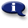# .

## Syllabus Information

Spring 2022
Dec 03,2023Use this page to maintain syllabus information, learning objectives, required materials, and technical requirements for the course.

Syllabus Information
MTH 070 - Introductory Algebra
Associated Term: Spring 2022
Learning Objectives: Upon successful completion of this course, the student should be able to: 1. Maintain, use, and expand skills and concepts learned in previous mathematics courses. Perform operations with fractions and decimals. Use unit analysis to convert units and solve problems. 2. Perform addition, subtraction, multiplication, and division of rational numbers. 3. Use and apply the concepts and language of algebraic expressions. Use variables to construct algebraic expressions. Evaluate algebraic expressions and simplify expressions using order of operations. Simplify algebraic expressions by removing parentheses and combining like terms. 4. Solve linear equations and formulas algebraically. Solve linear inequalities, graph their solutions on a number line, and use interval notation. 5. Use algebra to solve application problems. Translate verbal models into algebraic expressions and/or equations to solve problems. Solve problems using ratios and proportions. Solve problems involving similar triangles. Solve geometry problems involving perimeter, area, and volume. 6. Interpret information, represented numerically and graphically, and recognize linear relationships represented verbally, numerically, graphically, and algebraically. Read and interpret information given in a table or from a graph. Locate points in a rectangular coordinate system and represent equations in two variables graphically. Identify the horizontal and vertical intercepts of the graph of an equation and interpret them in terms of an application. Identify the slope of a line and interpret it in terms of an application. Use the slope-intercept form of the equation of a line. Use the point-slope form of the equation of a line. Recognize the relationship between the slopes of two lines that are parallel or perpendicular. 7. Solve linear systems of two equations algebraically and graphically. Solve application problems involving linear systems of equations (including simple interest, motion, and mixture problems.) 8. Evaluate and/or simplify expressions using the rules of exponents. 9. Use scientific notation. 10. Recognize and use the terminology of polynomials. Evaluate polynomials. Add, subtract, multiply, and divide polynomials (by a monomial). 11. Factor polynomials, including multivariable polynomials. Factor polynomials by removing a common monomial factor. Factor polynomials by grouping. Factor trinomials. Factor special products. 12. Recognize and use quadratic equations. Sketch the graph of a quadratic equation in two variables and identify the intercepts and the vertex graphically. Solve a quadratic equation by factoring. Solve applications by writing and solving quadratic equations (by factoring), including problems involving the Pythagorean Theorem. 13. Recognize and use rational expressions. Recognize values of a variable that make a rational expression undefined. Reduce rational expressions to lowest terms. Multiply and divide rational expressions. Add and subtract rational expressions with like denominators. Find the least common denominator of two or more rational expressions. Add and subtract rational expressions with unlike denominators. 14. Make appropriate and efficient use of a scientific calculator.
Required Materials:
Technical Requirements: